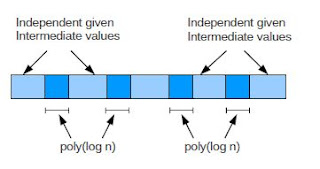Eretz Yisrael Time

Wednesday, August 11, 2010
There you have it. P!=NP.

In cryptology class, one of the first problems discussed is, is P=NP (or P!=NP).

What it means in English is, can a difficult equation that can be verified by a computer in short period of time, also be solved by a computer in a short period of time.In real world terms for example, one question this problem is asking is, can an encrypted message that can be verified as accurate, also be cracked in a short time.

The answer to this is important. If P=NP then eventually we will be able to find quick solutions to any encrypted message - and many of our current encryption methods would need to be replaced.

If the answer is no, then it is possible to create ciphers, such that deciphering them is guaranteed to take a very, very long time.

Consider a jigsaw puzzle. You can look at it and quickly see that it is solved. But it takes time to actually solve it. But need that be so (metaphorically speaking)?There are of course other applications to this problem.

The P vs NP problem is considered so difficult that it is one of seven problems that the Clay Mathematics Institute is giving away \$1,000,000 to solve.

Hewlett-Packard Research Laboratory researcher Vinay Deolalikar in India (hence the Taj Mahal above) is claiming to have solved the P=NP problem in a 100 page document.

He says P!=NP.

The document is currently undergoing peer review.